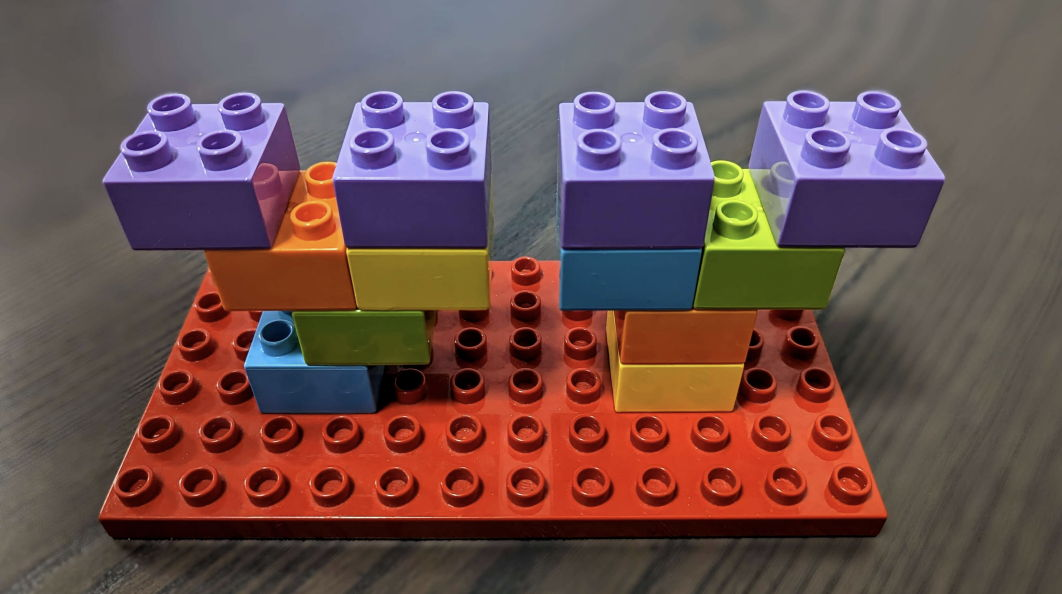시간 제한메모리 제한제출정답맞힌 사람정답 비율
2 초 1024 MB222100.000%

## 문제

Vittorio is playing with his new LEGO Duplo bricks. All the bricks have the shape of a square cuboid with a $2 \times 2$ square base and a height of $1$. They can be arranged in the 3D space to build structures, provided that the following rules are met:

1. No two bricks can intersect, but they can touch on their faces.
2. The corners of every brick must have integer coordinates (so bricks are axis-aligned) and the $z$ coordinates of all corners must be non-negative.
3. The square bases of every brick must be parallel to the ground (i.e. the plane $z = 0$).
4. The lower base of any brick that is not touching the ground must touch the upper base of some other brick in a region of positive area (when this happens, the two bricks stay attached to each other thanks to small studs).

For example, this is a valid structure:Vittorio wants to build a structure that includes purple bricks in the following $n$ positions: $(x_1, 0, h), (x_2, 0, h), \dots , (x_n, 0, h)$ — these are the coordinates of the centers of their lower bases; note that all of these bricks have $y$ coordinate equal to $0$ and $z$ coordinate equal to $h$. Vittorio will use additional bricks of other colors to support the purple bricks. He is willing to place bricks only in positions where the center of the lower base has $y$ coordinate equal to $0$. What is the minimum number of additional bricks needed?

It can be shown that a valid construction always exists.

## 입력

The first line contains two integers $n$ and $h$ ($1 ≤ n ≤ 300$, $0 ≤ h ≤ 10^9$) — the number of purple bricks and their common $z$ coordinate.

The second line contains $n$ integers $x_1, x_2, \dots , x_n$ ($1 ≤ x_i ≤ 10^9$, $x_i+1 < x_{i+1}$) — the $x$ coordinates of the purple bricks (centers of the bases), given in increasing order.

## 출력

Print the minimum number of additional bricks needed.

## 예제 입력 1

4 0
2 7 11 13


## 예제 출력 1

0


All the purple bricks lie on the ground, so no additional bricks are needed.

## 예제 입력 2

4 1
2 7 11 13


## 예제 출력 2

3


Vittorio will have to place supporting bricks under the purple bricks, and he can use a single brick to support both the third and the fourth purple bricks. For example, he can place additional bricks at positions $(3, 0, 0), (7, 0, 0)$ and $(12, 0, 0)$. It can be shown that it is impossible to build a valid construction using less than $3$ additional bricks.

## 예제 입력 3

4 100
2 7 11 13


## 예제 출력 3

107


## 예제 입력 4

4 3
2 5 8 11


## 예제 출력 4

8


A possible structure that minimizes the number of additional bricks is shown in the problem description.